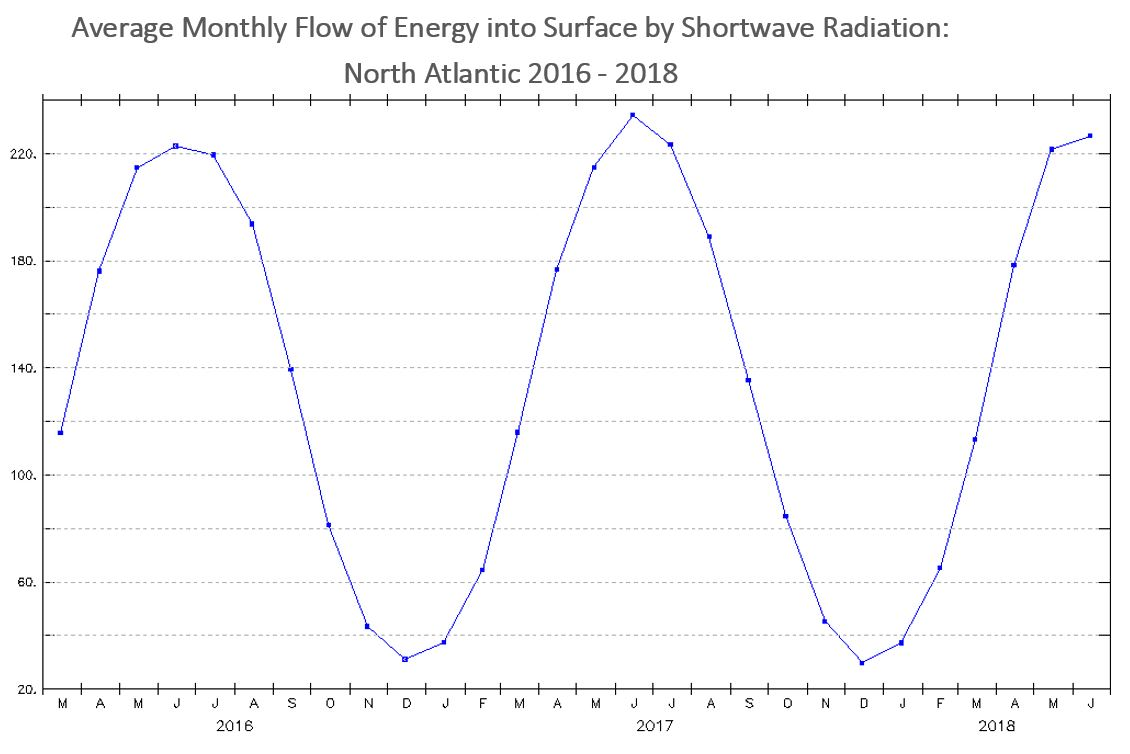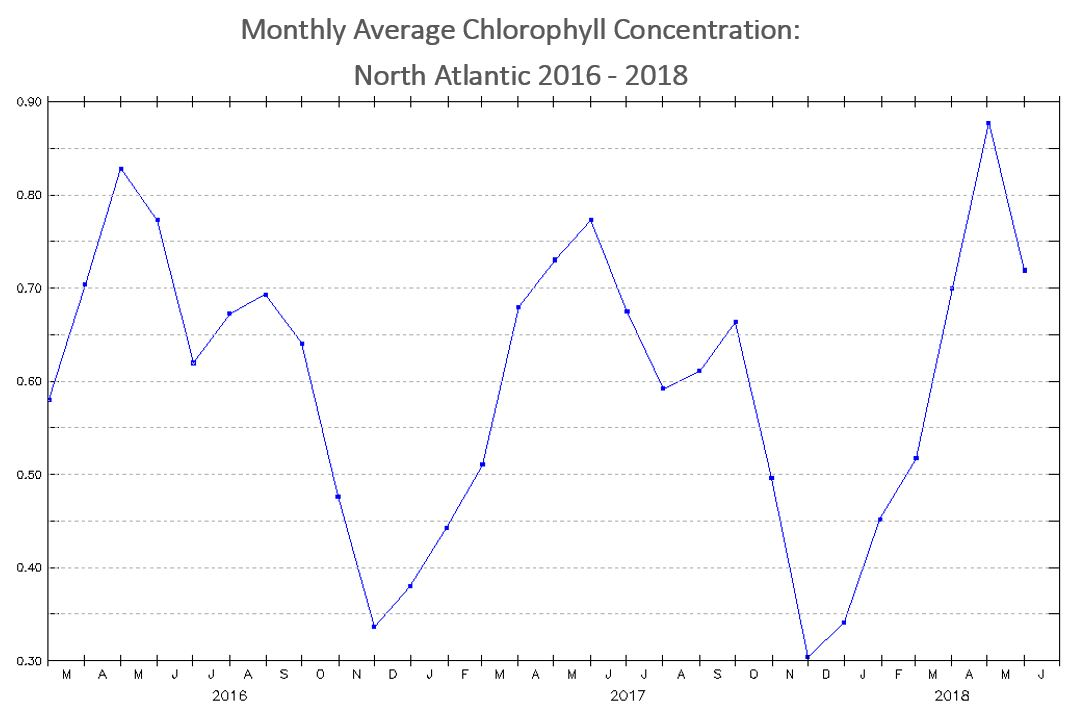# Analyzing Seasonal Phytoplankton & Energy Flow: Student Activity

### Student Directions

Analyze the line plot displaying Monthly Flow of Energy into Surface by Shortwave Radiation between the years of 2016 and 2018 in the North Atlantic Ocean and then answer the following questions.

The flow of energy into surface by shortwave radiation represents visible light coming from the Sun. The units of these data Watts per square meter, which is the flow of energy spread out over an areaFive Watts per square meter is equivalent to the power used by a standard cell phone charger (5 Watts) passing through a square piece of paper with length and width of 1 meter.

1. What variable is represented on the x-axis? What is the range of values?
2. What variable is represented on the y-axis? What is the range of values?
3. Describe the pattern that is revealed over the three years.
4. Make a prediction about what chlorophyll data collected over the same location in the North Atlantic over 2016-2018 will show.Analyze the graph displaying Monthly Average Chlorophyll Concentration between the years of 2016 and 2018 in the North Atlantic Ocean and then answer the following questions. The units for chlorophyll concentration in this graph is milligrams of chlorophyll per cubic meter of seawater. This is a very small mass unit. To compare, the average mass of a feather from a chicken is about 8 milligrams.1. What variable is represented on the x-axis? What is the range of values?
2. What variable is represented on the y-axis? What is the range of values?
3. Describe the pattern that is revealed over the three years.
4. Look for evidence in the data that supports or refutes your prediction.
5. Compare the two line graphs.  Describe what these graphs have in common?  How are they different?
6. Use scientific reasoning to identify different factors that may contribute to these similarities.
7. Brainstorm the factors that may contribute to their differences.

### Teacher Note

Teachers, these mini lessons/student activities are perfect "warm up" tasks that can be used as a hook, bellringer, exit slip, etc.

1. The link to the school/institution’s teacher directory where you are employed so we can verify that you are a teacher
2. Ensure that the school email address is provided in your response as we are unable to send to personal email accounts